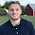Java program to find average of N number

Java program to find average

Java program to find average of 5 number

Java program to find average of 10 number

Java program to find average without using array

Program:-

import java.util.Scanner;
class group{
public static void main(String arng[]){
Scanner data = new Scanner(System.in);
int i, num, temp = 0;
float sum = 0;
System.out.print("How may numbers you want take average: ");
num=data.nextInt();
System.out.println("Enter " +num+ " for average");
for(i=0; i < num; i++)
{
temp = data.nextInt();
sum = sum + temp;
}
sum = sum/num;
System.out.println("Average of " +num+ " is "+sum);
}
}

Output:-

How may numbers you want take average: 4
Enter 4 for average
5
6
7
8
Average of 4 is 6.5

Learn Java:-
Java codes

Java Programs:-
List of Java Programs

One Comment

1.could you show how to find average of n numbers and then find the numbers above the average?